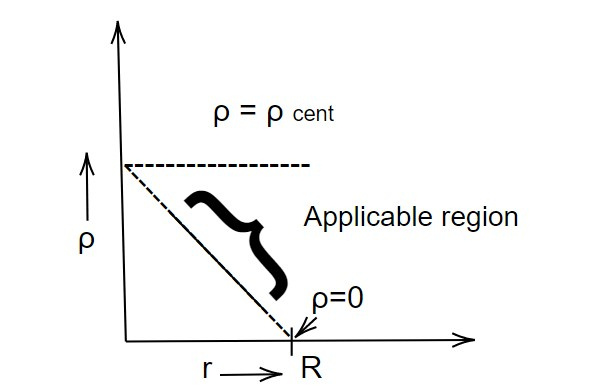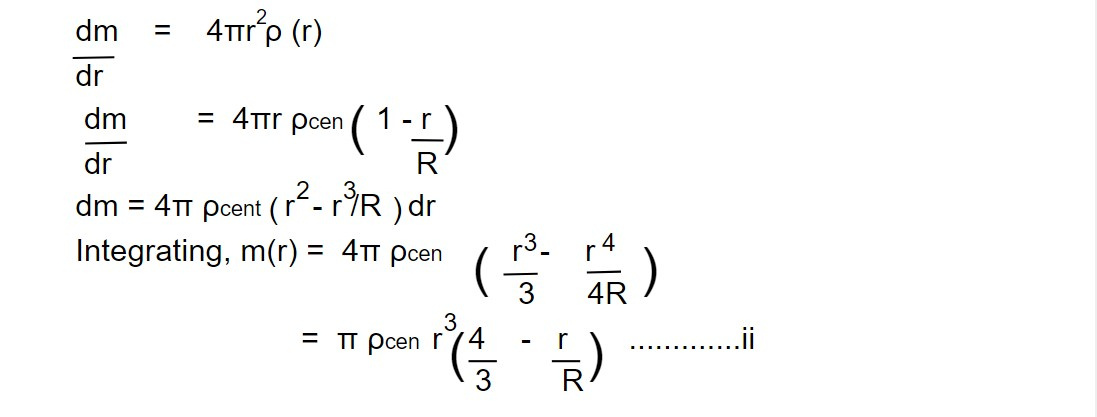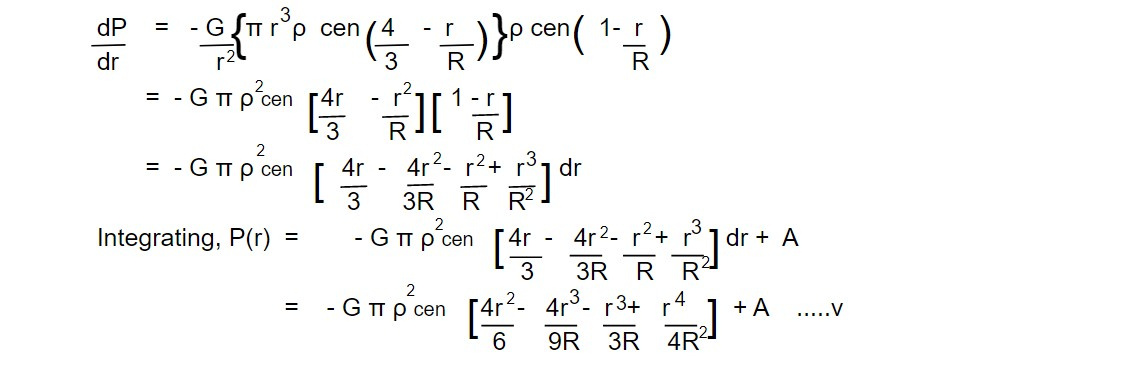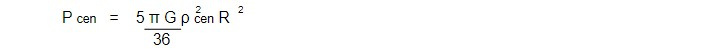# Linear Stellar Model

The Linear Stellar Model is a mathematical model used to describe the dynamics of a large number of stars in a galaxy. It uses linear algebra and linear dynamical systems to describe the motion of stars under the influence of gravitational forces. It assumes that the stars move in straight lines and that their velocity is constant, which makes it a simple and efficient way to model the dynamics of a large number of stars. Linear stellar models are useful in astrophysics for several reasons:

1. Understanding star formation and evolution: Linear models can be used to study the physical processes that govern the formation and evolution of stars. These models help us understand how a star changes over time, including its internal structure, temperature, luminosity, and other important characteristics.

2. Predicting observables: By solving the equations that govern the structure of a star, linear models can be used to make predictions about observable properties of stars, such as their brightness, spectra, and distances. These predictions can be compared to observations to validate or refine our understanding of the star.

3. Characterizing exoplanets: Linear models can be used to study the properties of exoplanets, including their masses, radii, and orbits. This information can be used to understand the formation and evolution of exoplanetary systems, and to search for potentially habitable worlds.

4. Studying extreme objects: Linear models can be used to study extreme objects, such as black holes, neutron stars, and white dwarfs. These objects have extremely high densities and pressures, and understanding their properties is important for our overall understanding of the universe.

In summary, linear stellar models are useful tools for astrophysics research, allowing us to make predictions and gain insights into a wide range of astronomical objects and phenomena.

The figurative derivation for linear stellar model is described asAccording to linear stellar model, density varies linearly asHere, we have assumed that the density at the central region is maximum and at the surface is zero.

Now we use mass continuity relation i.eWe normalize this equation in order to get total mass of the star.

At r=R, m(r) = M

Thus equation (ii) takes a form,Here, eq ii is named as mass profile. This equation is fourth order polynomial with no slope. This means mass do not vary linearly or no directional is expected. The variation of mass with r is random (monotonically change with given changing slopes).

Now, we use hydrostatic equilibrium to find the pressure profile,Substituting mass and density profile from equation ii and i.Now we need to find out the value of A. For this, we need the hypothesis of linear stellar model i.e

ρ surface (r= R) =0, this implies

Thus equation v takes the formSubstituting the value of A in equation v, we get pressure profile i.eThis is our expression for pressure profile. This is fourth order polynomial having three changing slopes with no constant slope. Thus simplify for the mass profile, pressure runs with 'r' randomly. Thus, in a linear stellar model, the pressure profile is described by the equation of state, which relates the pressure to the density of the star. The pressure profile in a star can have a significant impact on its structure and evolution, and is an important aspect of astrophysical research.

Hence, the randomness increases than that of the mass profile. Now, we calculate central pressure by putting r=0, i.eUsing equation iv.,Here we can write the central pressure is ten times larger than that of the limiting central pressure. It suggests that the star is close to collapsing. This can be due to a number of factors, such as the exhaustion of nuclear fuel in the center, which would cause the core to contract and the pressure to increase. If the central pressure exceeds the limiting central pressure, the star will undergo gravitational collapse, possibly leading to a supernova explosion or the formation of a compact object like a neutron star or black hole.

In summary, a central pressure that is ten times larger than the limiting central pressure is an indication that a star is near the brink of collapse, and its future evolution will depend on a number of physical processes and factors.

This note is taken from Astrophysics, MSC Nepal.

Previous note: Hydrostatic equilibrium

This note is a part of the Physics Repository.